# VBA MS Access Form Filter ExampleThis post is going to show you a ms access form filter sample.

The database I created only has one table called “Categories”.

We are going to have a form called “frmCategory”.The form is going to be a “Split Form”, because that seems to be quicker than the “main form / sub form” setup.

The “Datasheet on Bottom” setting is set giving it this effect. Having this format, gives us this effect: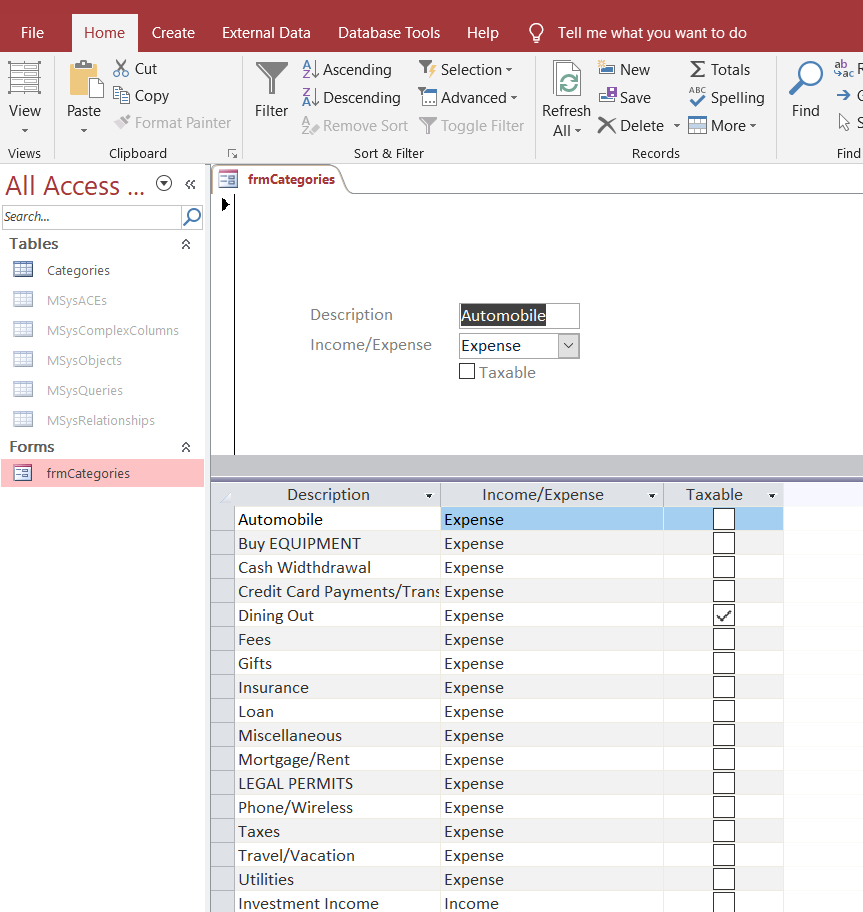These are the settings for the “Split Form”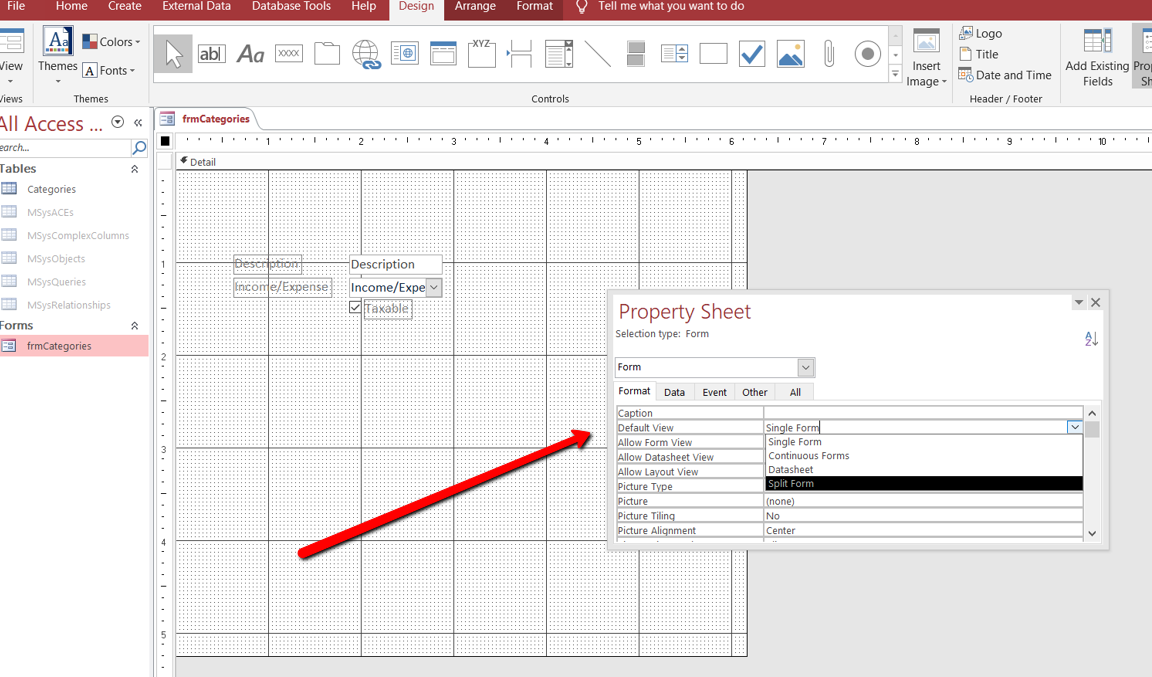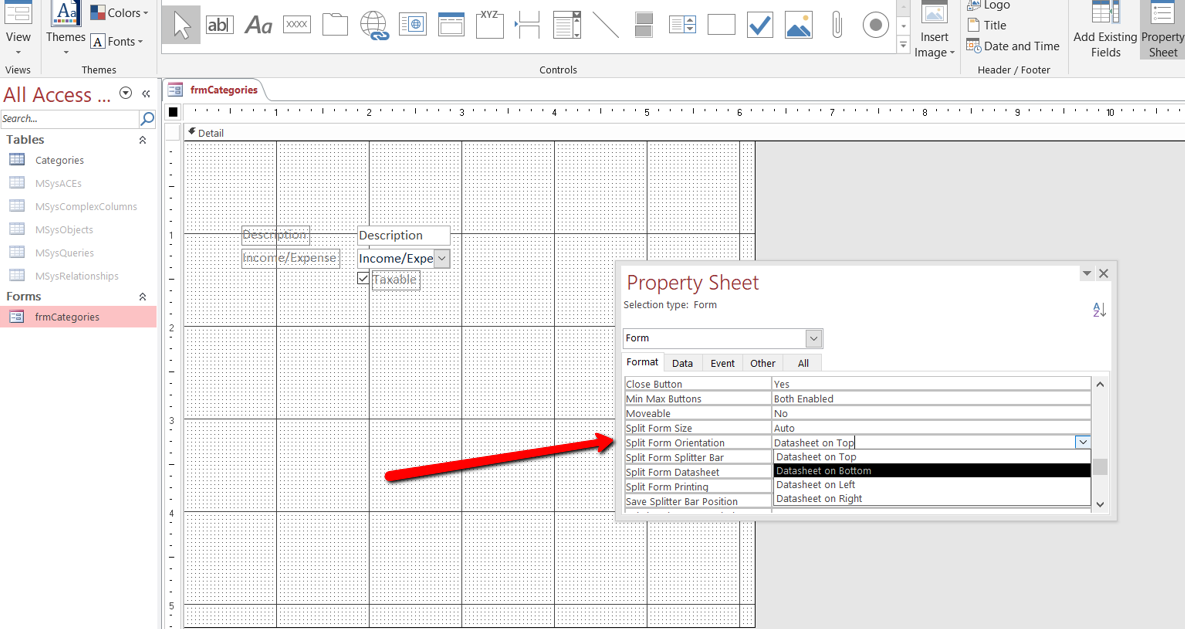I reordered my fields so I can filter better (here is how it looks):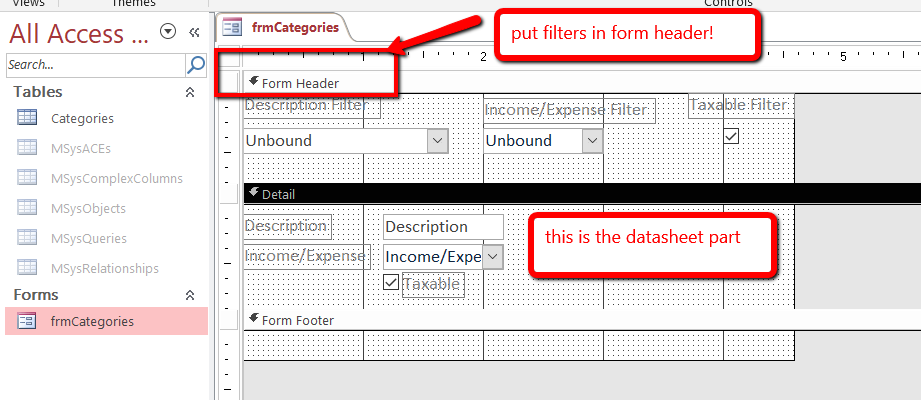Here is my form all together: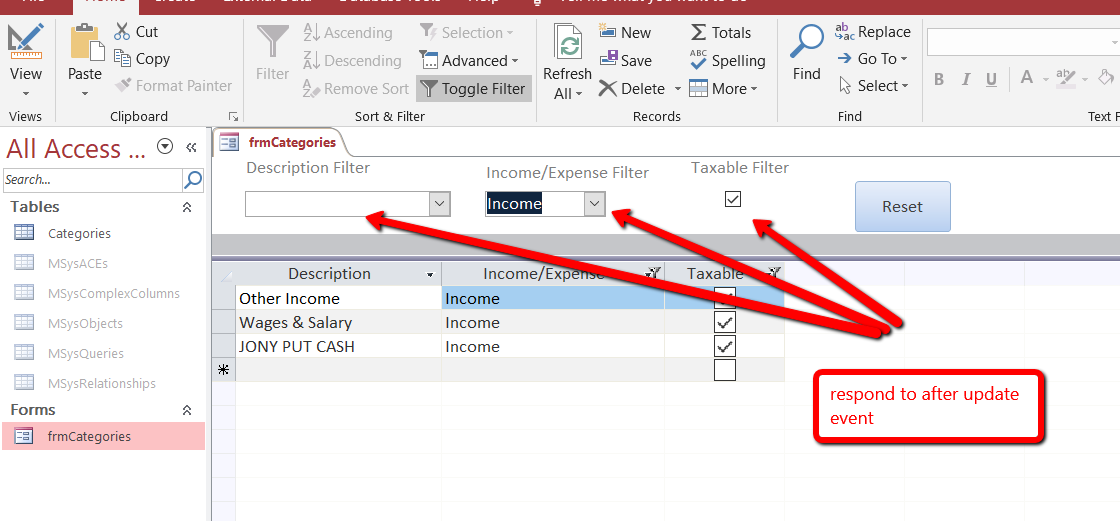…and here is my code:

```
123456789101112131415161718192021222324252627282930313233343536373839404142434445Option Compare Database

Private m_Where As String

Private Sub btnReset_Click()
Me.FilterOn = False

'clear all the variables
m_Where = ""
Me.cboDescription = ""
Me.cboIncomeExpense = ""
Me.chkTax = 0

End Sub

Private Sub cboDescription_AfterUpdate()

m_Where = m_Where &amp; " AND Description = '" &amp; Me.cboDescription &amp; "'"
FilterThis

End Sub

Private Sub cboIncomeExpense_AfterUpdate()

m_Where = m_Where &amp; " AND [Income/Expense] = '" &amp; Me.cboIncomeExpense &amp; "'"
FilterThis
End Sub
Private Sub chkTax_AfterUpdate()
m_Where = m_Where &amp; " AND [Taxable] = " &amp; Me.chkTax
FilterThis
End Sub

Sub FilterThis()

'clean the string first
If Left(m_Where, 4) = " AND" Then
m_Where = Mid(m_Where, 5)
End If

Me.Filter = m_Where
Me.FilterOn = True

End Sub

```

The variable “m_Where” is where the filter string is stored, and has modular level scope, so the value persists while the form is open.

Let me know if you have any questions.#### “Go Fund Me” Page(\$5 suggested amount)## Free! Subscribe To Our YouTube Channel!